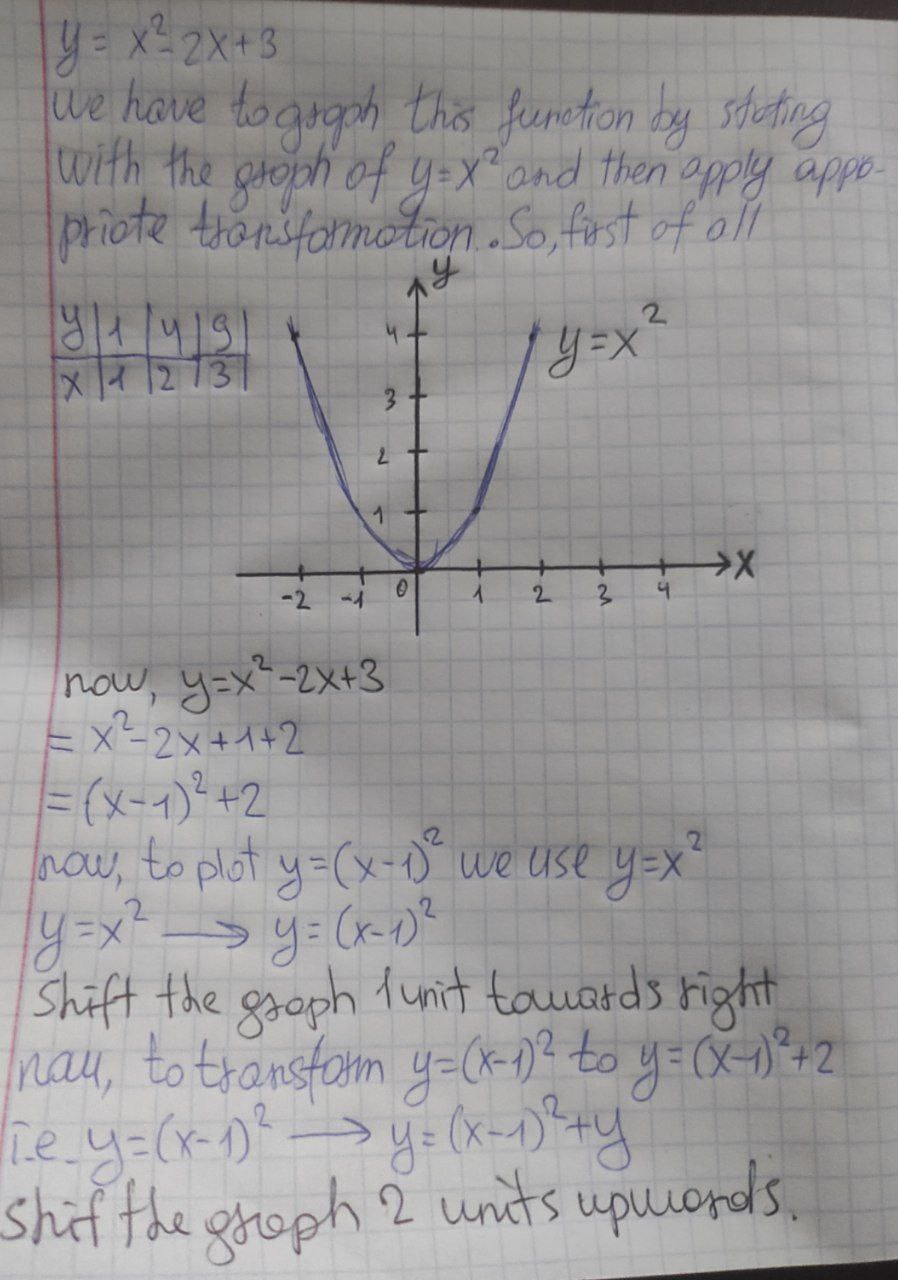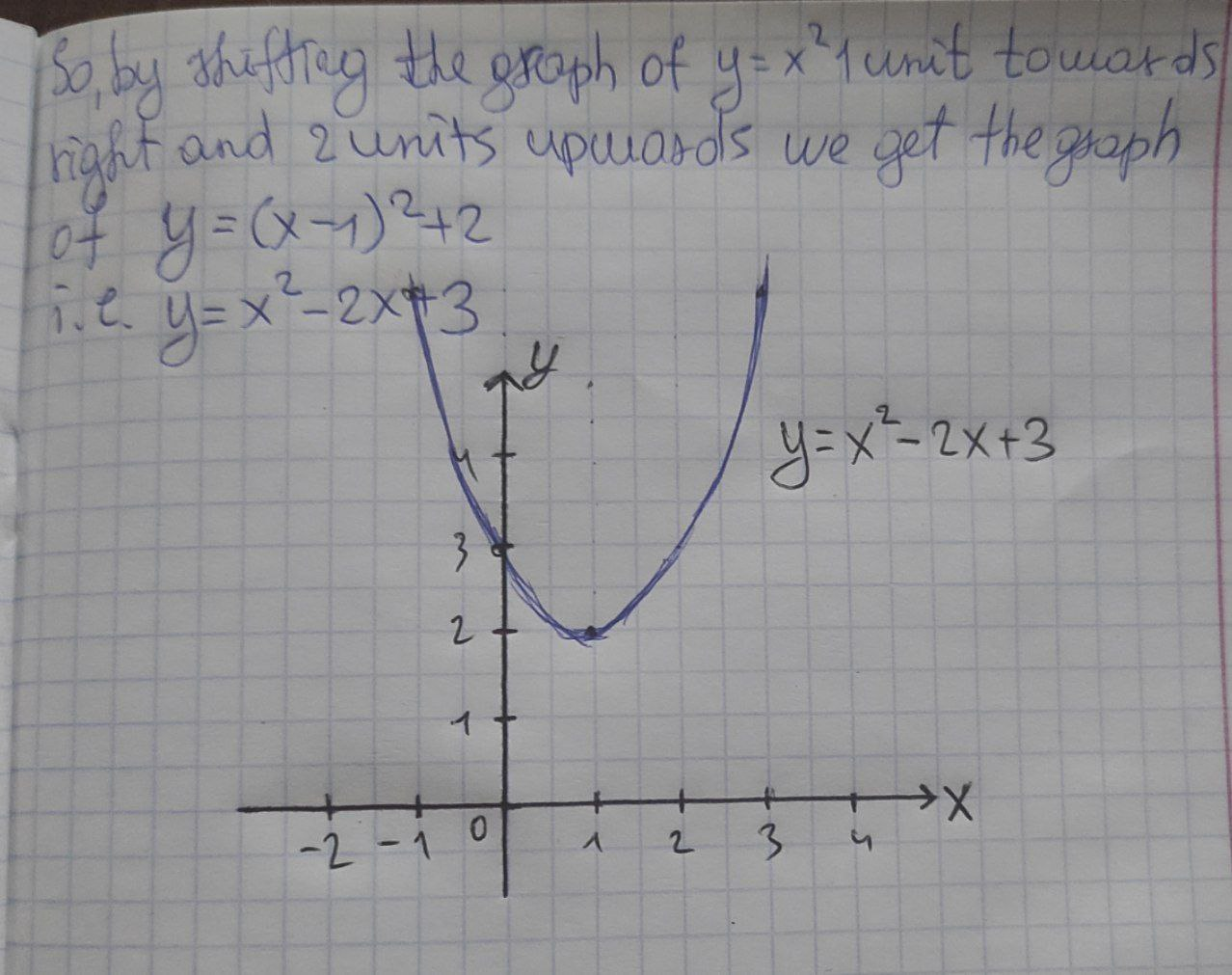2022-03-07

)Graph the function y = x^2− 2x + 3, not by plotting points, but by starting with the graph of y = x^2 and then applying the appropriate transformations. Describe your transformations